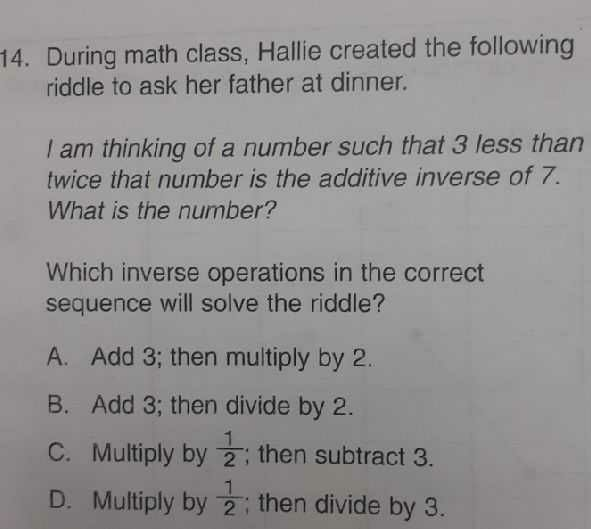### ¿Todavía tienes preguntas de matemáticas?

Pregunte a nuestros tutores expertos
Algebra
Pregunta14. During math class, Hallie created the following riddle to ask her father at dinner. I am thinking of a number such that $$3$$ less than twice that number is the additive inverse of $$7$$ . What is the number? Which inverse operations in the correct sequence will solve the riddle? A. Add $$3 ;$$ then multiply by $$2$$ . B. Add 3; then divide by $$2$$ . C. Multiply by $$\frac { 1 } { 2 }$$ ; then subtract $$3 .$$ D. Multiply by $$\frac { 1 } { 2 }$$ ; then divide by $$3$$ .